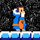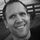cancel
Showing results for
Did you mean:Helper IV

## "IN" operator doesn't work

HI,

How to write following code in dax Measure ?

if ( Column1 in ("Value1","Value2"...."ValueN") then [Revenue] else 0)

I am struggling here: I am able to give it like this

if ( Column1 ="Value1" or Column1 ="Value2".... or Column1 ="ValueN") then [Revenue] else 0)

which is not efficient...

I need  calculate( sum(FactT[revenue]), filter(FactT,FactT[Column1]  in ("Value1","Value2"...."Value3")))

is there any function that i can use... instead of 'IN' operator...??

1 ACCEPTED SOLUTIONFrequent Visitor

## Hi,

The SQL functions IN is useful to implement tests over a set of values. This article describes the corresponding syntax in DAX language.

## Implementing IN as nested OR conditions

Consider the following query:

 123 SELECT DISTINCT EnglishCountryRegionNameFROM DimGeographyWHERE CountryRegionCode IN ('US', 'CA', 'AU' )

In DAX there are no operators that corresponds to the IN available in SQL. Thus, you have to write a list of corresponding nested OR functions:

 1234567891011 EVALUATECALCULATETABLE (    VALUES ( Geography[Country Region Name] ),    OR (        OR (            Geography[Country Region Code] = "US",            Geography[Country Region Code] = "CA"        ),        Geography[Country Region Code] = "AU"    ))

As an alternative, you can use the logical OR operator (||):

 1234567 EVALUATECALCULATETABLE (    VALUES ( Geography[Country Region Name] ),    Geography[Country Region Code] = "US"    || Geography[Country Region Code] = "CA"    || Geography[Country Region Code] = "AU")

This DAX syntax could be a real issue when the list of values to test is long, because the length of the query string might become unmanageable. At that point, a possible alternative is storing the list of values in a separate table, similar to the one called Selection in the following example:

 123456789101112 EVALUATECALCULATETABLE (    VALUES ( Geography[Country Region Name] ),    FILTER (        ALL ( Geography[Country Region Code] ),        CONTAINS (            VALUES ( Selection[Country Region Code] ),                Selection[Country Region Code],            Geography[Country Region Code]        )    ))
3 REPLIES 3Frequent Visitor

## Hi,

The SQL functions IN is useful to implement tests over a set of values. This article describes the corresponding syntax in DAX language.

## Implementing IN as nested OR conditions

Consider the following query:

 123 SELECT DISTINCT EnglishCountryRegionNameFROM DimGeographyWHERE CountryRegionCode IN ('US', 'CA', 'AU' )

In DAX there are no operators that corresponds to the IN available in SQL. Thus, you have to write a list of corresponding nested OR functions:

 1234567891011 EVALUATECALCULATETABLE (    VALUES ( Geography[Country Region Name] ),    OR (        OR (            Geography[Country Region Code] = "US",            Geography[Country Region Code] = "CA"        ),        Geography[Country Region Code] = "AU"    ))

As an alternative, you can use the logical OR operator (||):

 1234567 EVALUATECALCULATETABLE (    VALUES ( Geography[Country Region Name] ),    Geography[Country Region Code] = "US"    || Geography[Country Region Code] = "CA"    || Geography[Country Region Code] = "AU")

This DAX syntax could be a real issue when the list of values to test is long, because the length of the query string might become unmanageable. At that point, a possible alternative is storing the list of values in a separate table, similar to the one called Selection in the following example:

 123456789101112 EVALUATECALCULATETABLE (    VALUES ( Geography[Country Region Name] ),    FILTER (        ALL ( Geography[Country Region Code] ),        CONTAINS (            VALUES ( Selection[Country Region Code] ),                Selection[Country Region Code],            Geography[Country Region Code]        )    ))Super User

How about Power Query "M", can I have something similar to "In" operator with it?

If this post helps, then please consider Accept it as the solution to help the other members find it more quickly.

Happy to help!I don't know if this part of the forum is actually meant as a help section, but could you provide a bit example data on the issue?

Im wondering if Value 1,2 etc are values inside the column or actual measure values 🙂